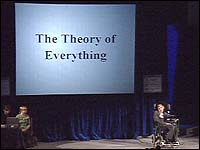﻿ Tourgueniev ce hérosIf space and imaginary time are indeed like the surface of the Earth, there wouldn’t be any singularities in the imaginary time direction, at which the laws of physics would break down. And there wouldn’t be any boundaries, to the imaginary time space-time, just as there aren’t any boundaries to the surface of the Earth. This absence of boundaries means that the laws of physics would determine the state of the universe uniquely, in imaginary time. But if one knows the state of the universe in imaginary time, one can calculate the state of the universe in real time. One would still expect some sort of Big Bang singularity in real time. So real time would still have a beginning. But one wouldn’t have to appeal to something outside the universe, to determine how the universe began. Instead, the way the universe started out at the Big Bang would be determined by the state of the universe in imaginary time. Thus, the universe would be a completely self-contained system. It would not be determined by anything outside the physical universe, that we observe. Other scientists were much more ready than Einstein to modify the classical 19th century view of determinism. A new theory, called quantum mechanics, was put forward by Heisenberg, the Austrian, Erwin Schroedinger, and the British physicist, Paul Dirac. Dirac was my predecessor but one, as the Lucasian Professor in Cambridge. Although quantum mechanics has been around for nearly 70 years, it is still not generally understood or appreciated, even by those that use it to do calculations. Yet it should concern us all, because it is a completely different picture of the physical universe, and of reality itself. In quantum mechanics, particles don’t have well defined positions and speeds. Instead, they are represented by what is called a wave function.This is a number at each point of space. The size of the wave function gives the probability that the particle will be found in that position. The rate, at which the wave function varies from point to point, gives the speed of the particle. One can have a wave function that is very strongly peaked in a small region. This will mean that the uncertainty in the position is small. But the wave function will vary very rapidly near the peak, up on one side, and down on the other. Thus the uncertainty in the speed will be large. Similarly, one can have wave functions where the uncertainty in the speed is small, but the uncertainty in the position is large. So three dimensions, seems to be the minimum for life. But just as one can think of two dimensional beings living on the surface of the Earth, so one could imagine that the three dimensional space in which we live, was the surface of a sphere, in another dimension that we don’t see. If the sphere were very large, space would be nearly flat, and Euclidean geometry would be a very good approximation over small distances. But we would notice that Euclidean geometry broke down, over large distances. As an illustration of this, imagine a team of painters, adding paint to the surface of a large ball. As the thickness of the paint layer increased, the surface area would go up. If the ball were in a flat three-dimensional space, one could go on adding paint indefinitely, and the ball would get bigger and bigger. However, if the three-dimensional space, were really the surface of a sphere in another dimension, its volume would be large but finite. As one added more layers of paint, the ball would eventually fill half the space. After that, the painters would find that they were trapped in a region of ever decreasing size, and almost the whole of space, was occupied by the ball, and its layers of paint. So they would know that they were living in a curved space, and not a flat one. This example shows that one can not deduce the geometry of the world from first principles, as the ancient Greeks thought. Instead, one has to measure the space we live in, and find out its geometry by experiment. However, although a way to describe curved spaces, was developed by the German, George Friedrich Riemann, in 1854, it remained just a piece of mathematics for sixty years. It could describe curved spaces that existed in the abstract, but there seemed no reason why the physical space we lived in, should be curved. This came only in 1915, when Einstein put forward the General Theory of Relativity.
Old par igor le 13 Juin 2003